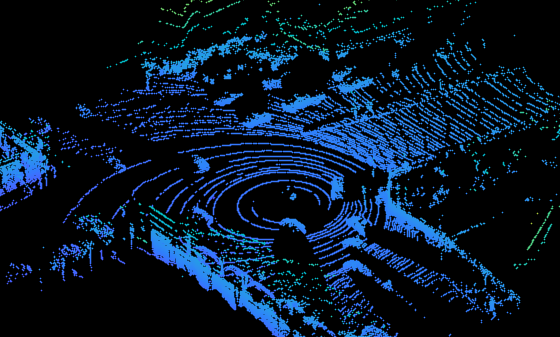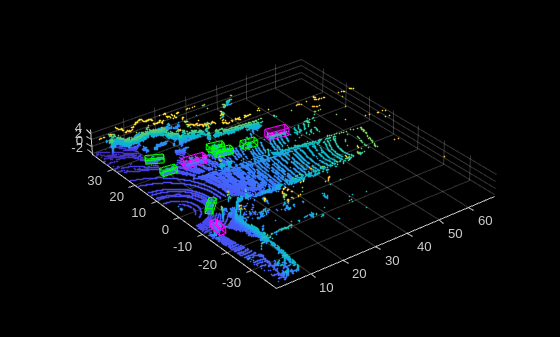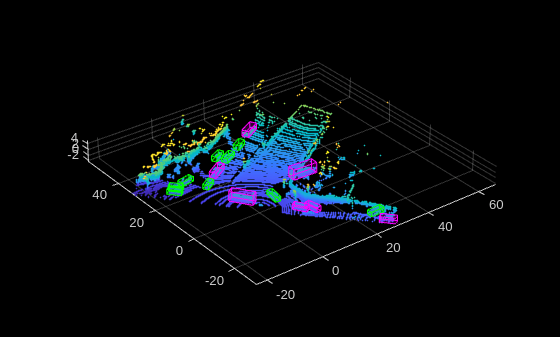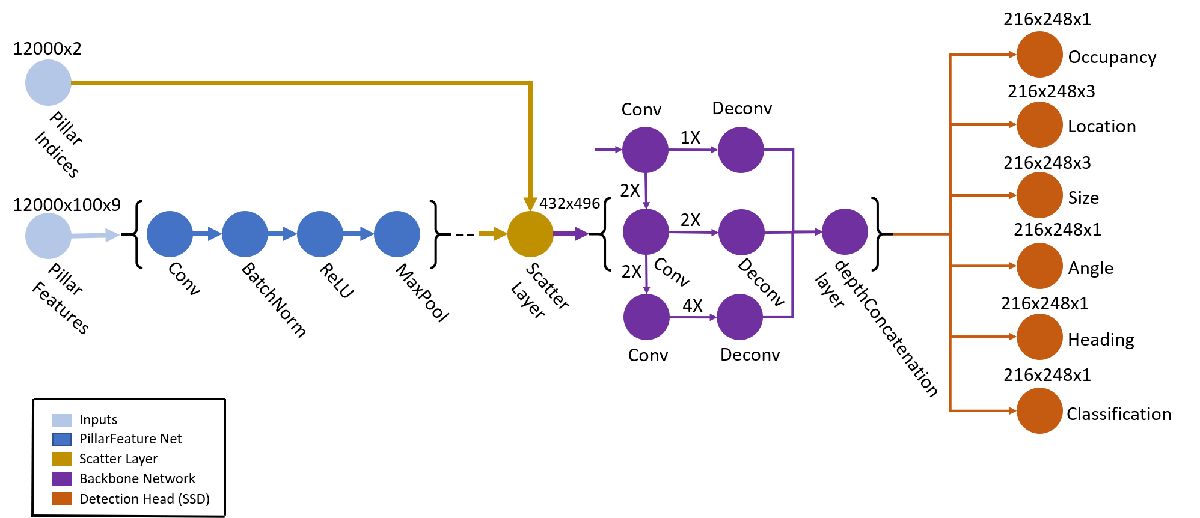# Lidar 3-D Object Detection Using PointPillars Deep Learning

This example shows how to train a PointPillars network for object detection in point clouds.

Lidar point cloud data can be acquired by a variety of lidar sensors, including Velodyne®, Pandar, and Ouster sensors. These sensors capture 3-D position information about objects in a scene, which is useful for many applications in autonomous driving and augmented reality. However, training robust detectors with point cloud data is challenging because of the sparsity of data per object, object occlusions, and sensor noise. Deep learning techniques have been shown to address many of these challenges by learning robust feature representations directly from point cloud data. One deep learning technique for 3-D object detection is PointPillars . Using a similar architecture to PointNet, the PointPillars network extracts dense, robust features from sparse point clouds called pillars, then uses a 2-D deep learning network with a modified SSD object detection network to estimate joint 3-D bounding boxes, orientations, and class predictions.

This example uses the PandaSet  data set from Hesai and Scale. PandaSet contains 8240 unorganized lidar point cloud scans of various city scenes captured using a Pandar64 sensor. The data set provides 3-D bounding box labels for 18 different object classes, including car, truck, and pedestrian.

This example uses a subset of PandaSet that contains 2560 preprocessed organized point clouds. Each point cloud covers ${360}^{\mathit{o}}$ of view, and is specified as a 64-by-1856 matrix. The point clouds are stored in PCD format and their corresponding ground truth data is stored in the `PandaSetLidarGroundTruth.mat` file. The file contains 3-D bounding box information for three classes, which are car, truck, and pedestrian. The size of the data set is 5.2 GB.

Download the Pandaset dataset from the given URL using the `helperDownloadPandasetData` helper function, defined at the end of this example.

```outputFolder = fullfile(tempdir,'Pandaset'); lidarURL = ['https://ssd.mathworks.com/supportfiles/lidar/data/' ... 'Pandaset_LidarData.tar.gz']; helperDownloadPandasetData(outputFolder,lidarURL);```

Depending on your Internet connection, the download process can take some time. The code suspends MATLAB® execution until the download process is complete. Alternatively, you can download the data set to your local disk using your web browser and extract the file. If you do so, change the `outputFolder` variable in the code to the location of the downloaded file.

Download the pretrained network from the given URL using the `helper`D`ownloadPretrainedPointPillarsNet` helper function, defined at the end of this example. The pretrained model allows you to run the entire example without having to wait for training to complete. If you want to train the network, set the `doTraining` variable to `true`.

```pretrainedNetURL = ['https://ssd.mathworks.com/supportfiles/lidar/data/' ... 'trainedPointPillarsPandasetNet.zip']; doTraining = false; if ~doTraining helperDownloadPretrainedPointPillarsNet(outputFolder,pretrainedNetURL); end```

Create a file datastore to load the PCD files from the specified path using the `pcread` function.

```path = fullfile(outputFolder,'Lidar'); lidarData = fileDatastore(path,'ReadFcn',@(x) pcread(x));```

Load the 3-D bounding box labels of the car and truck objects.

```gtPath = fullfile(outputFolder,'Cuboids','PandaSetLidarGroundTruth.mat'); data = load(gtPath,'lidarGtLabels'); Labels = timetable2table(data.lidarGtLabels); boxLabels = Labels(:,2:3);```

Display the full-view point cloud.

```figure ptCld = read(lidarData); ax = pcshow(ptCld.Location); set(ax,'XLim',[-50 50],'YLim',[-40 40]); zoom(ax,2.5); axis off;``````reset(lidarData); ```

### Preprocess Data

The PandaSet data consists of full-view point clouds. For this example, crop the full-view point clouds to front-view point clouds using the standard parameters . These parameters determine the size of the input passed to the network. Selecting a smaller range of point clouds along the x, y, and z-axis helps detect objects that are closer to the origin and also decreases the overall training time of the network.

```xMin = 0.0; % Minimum value along X-axis. yMin = -39.68; % Minimum value along Y-axis. zMin = -5.0; % Minimum value along Z-axis. xMax = 69.12; % Maximum value along X-axis. yMax = 39.68; % Maximum value along Y-axis. zMax = 5.0; % Maximum value along Z-axis. xStep = 0.16; % Resolution along X-axis. yStep = 0.16; % Resolution along Y-axis. dsFactor = 2.0; % Downsampling factor. % Calculate the dimensions for the pseudo-image. Xn = round(((xMax - xMin) / xStep)); Yn = round(((yMax - yMin) / yStep)); % Define the pillar extraction parameters. gridParams = {{xMin,yMin,zMin},{xMax,yMax,zMax},{xStep,yStep,dsFactor},{Xn,Yn}};```

Use the c`ropFrontViewFromLidarData` helper function, attached to this example as a supporting file, to:

• Crop the front view from the input full-view point cloud.

• Select the box labels that are inside the ROI specified by `gridParams`.

```[croppedPointCloudObj,processedLabels] = cropFrontViewFromLidarData(... lidarData,boxLabels,gridParams);```
```Processing data 100% complete ```

Display the cropped point cloud and the ground truth box labels using the `helperDisplay3DBoxesOverlaidPointCloud` helper function defined at the end of the example.

```pc = croppedPointCloudObj{1,1}; gtLabelsCar = processedLabels.Car{1}; gtLabelsTruck = processedLabels.Truck{1}; helperDisplay3DBoxesOverlaidPointCloud(pc.Location,gtLabelsCar,... 'green',gtLabelsTruck,'magenta','Cropped Point Cloud');````reset(lidarData);`

### Create Datastore Objects for Training

Split the data set into training and test sets. Select 70% of the data for training the network and the rest for evaluation.

```rng(1); shuffledIndices = randperm(size(processedLabels,1)); idx = floor(0.7 * length(shuffledIndices)); trainData = croppedPointCloudObj(shuffledIndices(1:idx),:); testData = croppedPointCloudObj(shuffledIndices(idx+1:end),:); trainLabels = processedLabels(shuffledIndices(1:idx),:); testLabels = processedLabels(shuffledIndices(idx+1:end),:);```

So that you can easily access the datastores, save the training data as PCD files by using the `saveptCldToPCD` helper function, attached to this example as a supporting file. You can set `writeFiles` to "`false"` if your training data is saved in a folder and is supported by the `pcread` function.

```writeFiles = true; dataLocation = fullfile(outputFolder,'InputData'); [trainData,trainLabels] = saveptCldToPCD(trainData,trainLabels,... dataLocation,writeFiles);```
```Processing data 100% complete ```

Create a file datastore using `fileDatastore` to load PCD files using the `pcread` function.

`lds = fileDatastore(dataLocation,'ReadFcn',@(x) pcread(x));`

Createa box label datastore using `boxLabelDatastore` for loading the 3-D bounding box labels.

`bds = boxLabelDatastore(trainLabels);`

Use the `combine` function to combine the point clouds and 3-D bounding box labels into a single datastore for training.

`cds = combine(lds,bds);`

### Data Augmentation

This example uses ground truth data augmentation and several other global data augmentation techniques to add more variety to the training data and corresponding boxes. For more information on typical data augmentation techniques used in 3-D object detection workflows with lidar data, see Data Augmentations for Lidar Object Detection Using Deep Learning.

Read and display a point cloud before augmentation using the `helperDisplay3DBoxesOverlaidPointCloud` helper function, defined at the end of the example..

```augData = read(cds); augptCld = augData{1,1}; augLabels = augData{1,2}; augClass = augData{1,3}; labelsCar = augLabels(augClass=='Car',:); labelsTruck = augLabels(augClass=='Truck',:); helperDisplay3DBoxesOverlaidPointCloud(augptCld.Location,labelsCar,'green',... labelsTruck,'magenta','Before Data Augmentation');````reset(cds);`

Use the `generateGTDataForAugmentation` helper function, attached to this example as a supporting file, to extract all the ground truth bounding boxes from the training data.

`gtData = generateGTDataForAugmentation(trainData,trainLabels);`

Use the `groundTruthDataAugmentation` helper function, attached to this example as a supporting file, to randomly add a fixed number of car and truck class objects to every point cloud. Use the `transform` function to apply the ground truth and custom data augmentations to the training data.

```samplesToAdd = struct('Car',10,'Truck',10); cdsAugmented = transform(cds,@(x) groundTruthDataAugmenation(x,gtData,samplesToAdd));```

In addition, apply the following data augmentations to every point cloud.

• Random flipping along the x-axis

• Random scaling by 5 percent

• Random rotation along the z-axis from [-pi/4, pi/4]

• Random translation by [0.2, 0.2, 0.1] meters along the x-, y-, and z-axis respectively

`cdsAugmented = transform(cdsAugmented,@(x) augmentData(x));`

Display an augmented point cloud along with ground truth augmented boxes using the `helperDisplay3DBoxesOverlaidPointCloud` helper function, defined at the end of the example.

```augData = read(cdsAugmented); augptCld = augData{1,1}; augLabels = augData{1,2}; augClass = augData{1,3}; labelsCar = augLabels(augClass=='Car',:); labelsTruck = augLabels(augClass=='Truck',:); helperDisplay3DBoxesOverlaidPointCloud(augptCld(:,1:3),labelsCar,'green',... labelsTruck,'magenta','After Data Augmentation');````reset(cdsAugmented);`

### Extract Pillar Information

You can apply a 2-D convolution architecture to the point clouds for faster processing. To do so, first convert the 3-D point clouds to 2-D representation. Use the `transform` function with the `createPillars` helper function, attached to this example as a supporting file, to create pillar features and pillar indices from the point clouds. The helper function performs the following operations:

• Discretize 3-D point clouds into evenly spaced grids in the x-y plane to create a set of vertical columns called pillars.

• Select prominent pillars (P) based on the number of points per pillar (N).

• Compute the distance to the arithmetic mean of all points in the pillar.

• Compute the offset from the pillar center.

• Use the x, y, z location, intensity, distance, and offset values to create a nine dimensional (9-D) vector for each point in the pillar.

```% Define number of prominent pillars. P = 12000; % Define number of points per pillar. N = 100; cdsTransformed = transform(cdsAugmented,@(x) createPillars(x,gridParams,P,N));```

### Define Network

The PointPillars network uses a simplified version of the PointNet network that takes pillar features as input. For each pillar feature, the network applies a linear layer, followed by batch normalization and ReLU layers. Finally, the network applies a max-pooling operation over the channels to get high-level encoded features. These encoded features are scattered back to the original pillar locations to create a pseudo-image using the custom layer `helperscatterLayer`, attached to this example as a supporting file. The network then processes the pseudo-image with a 2-D convolutional backbone followed by various SSD detection heads to predict the 3-D bounding boxes along with its classes.Define the anchor box dimensions based on the classes to detect. Typically, these dimensions are the means of all the bounding box values in the training set . Alternatively, you can also use the `calculateAnchorBoxes` helper function, attached to the example, for obtaining the appropriate anchor boxes from any training set. The anchor boxes are defined in the format {length, width, height, z-center, yaw angle}.

```anchorBoxes = calculateAnchorsPointPillars(trainLabels); numAnchors = size(anchorBoxes,2); classNames = trainLabels.Properties.VariableNames; numClasses = numel(classNames);```

Next, create the PointPillars network using the `pointpillarNetwork` helper function, attached to this example as a supporting file.

`lgraph = pointpillarNetwork(numAnchors,gridParams,P,N,numClasses);`

### Specify Training Options

Specify the following training options.

• Set the number of epochs to 60.

• Set the mini-batch size to 2. You can set the mini-batch size to a higher value if you have more available memory.

• Set the learning rate to 0.0002.

• Set `learnRateDropPeriod` to 15. This parameter denotes the number of epochs after which to drop the learning rate based on the formula $\mathrm{learningRate}×\left(\mathrm{iteration}%\mathrm{learnRateDropPeriod}\right)×\mathrm{learnRateDropFactor}$.

• Set `learnRateDropFactor` to 0.8. This parameter denotes the rate by which to drop the learning rate after each `learnRateDropPeriod`.

• Set the gradient decay factor to 0.9.

• Set the squared gradient decay factor to 0.999.

• Initialize the average of gradients to [ ]. This is used by the Adam optimizer.

• Initialize the average of squared gradients to [ ]. This is used by the Adam optimizer.

```numEpochs = 60; miniBatchSize = 2; learningRate = 0.0002; learnRateDropPeriod = 15; learnRateDropFactor = 0.8; gradientDecayFactor = 0.9; squaredGradientDecayFactor = 0.999; trailingAvg = []; trailingAvgSq = [];```

### Train Model

Train the network using a CPU or GPU. Using a GPU requires Parallel Computing Toolbox™ and a CUDA® enabled NVIDIA® GPU. For more information, see GPU Support by Release (Parallel Computing Toolbox). To automatically detect if you have a GPU available, set `executionEnvironment` to `"auto"`. If you do not have a GPU, or do not want to use one for training, set `executionEnvironment` to `"cpu"`. To ensure the use of a GPU for training, set `executionEnvironment` to `"gpu"`.

Next, create a `minibatchqueue` (Deep Learning Toolbox) to load the data in batches of `miniBatchSize` during training.

```executionEnvironment = "auto"; if canUseParallelPool dispatchInBackground = true; else dispatchInBackground = false; end mbq = minibatchqueue(... cdsTransformed,3,... "MiniBatchSize",miniBatchSize,... "OutputEnvironment",executionEnvironment,... "MiniBatchFcn",@(features,indices,boxes,labels) ... helperCreateBatchData(features,indices,boxes,labels,classNames),... "MiniBatchFormat",["SSCB","SSCB",""],... "DispatchInBackground",true);```

To train the network with a custom training loop and enable automatic differentiation, convert the layer graph to a `dlnetwork` (Deep Learning Toolbox) object. Then create the training progress plotter using the `helperConfigureTrainingProgressPlotter` helper function, defined at the end of this example.

Finally, specify the custom training loop. For each iteration:

• Read the point clouds and ground truth boxes from the `minibatchqueue` (Deep Learning Toolbox) object using the `next` (Deep Learning Toolbox) function.

• Evaluate the model gradients using `dlfeval` (Deep Learning Toolbox) and the `modelGradients` function. The `modelGradients` helper function, defined at the end of example, returns the gradients of the loss with respect to the learnable parameters in `net`, the corresponding mini-batch loss, and the state of the current batch.

• Update the network parameters using the `adamupdate` (Deep Learning Toolbox) function.

• Update the state parameters of `net`.

• Update the training progress plot.

```if doTraining % Convert layer graph to dlnetwork. net = dlnetwork(lgraph); % Initialize plot. fig = figure; lossPlotter = helperConfigureTrainingProgressPlotter(fig); iteration = 0; % Custom training loop. for epoch = 1:numEpochs % Reset datastore. reset(mbq); while(hasdata(mbq)) iteration = iteration + 1; % Read batch of data. [pillarFeatures,pillarIndices,boxLabels] = next(mbq); % Evaluate the model gradients and loss using dlfeval % and the modelGradients function. [gradients,loss,state] = dlfeval(@modelGradients,net,... pillarFeatures,pillarIndices,boxLabels,gridParams,... anchorBoxes,numClasses,executionEnvironment); % Do not update the network learnable parameters if NaN values % are present in gradients or loss values. if helperCheckForNaN(gradients,loss) continue; end % Update the state parameters of dlnetwork. net.State = state; % Update the network learnable parameters using the Adam % optimizer. [net.Learnables,trailingAvg,trailingAvgSq] = ... adamupdate(net.Learnables,gradients,trailingAvg,... trailingAvgSq,iteration,learningRate,... gradientDecayFactor,squaredGradientDecayFactor); % Update training plot with new points. addpoints(lossPlotter,iteration,double(gather(extractdata(loss)))); title("Training Epoch " + epoch +" of " + numEpochs); drawnow; end % Update the learning rate after every learnRateDropPeriod. if mod(epoch,learnRateDropPeriod) == 0 learningRate = learningRate * learnRateDropFactor; end end else preTrainedMATFile = fullfile(outputFolder,'trainedPointPillarsPandasetNet.mat'); pretrainedNetwork = load(preTrainedMATFile,'net'); net = pretrainedNetwork.net; end```

### Generate Detections

Use the trained network to detect objects in the test data:

• Read the point cloud from the test data.

• Use the `generatePointPillarDetections` helper function, attached to this example as a supporting file, to get the predicted bounding boxes and confidence scores.

• Display the point cloud with bounding boxes using the `helperDisplay3DBoxesOverlaidPointCloud` helper function, defined at the end of the example.

```ptCloud = testData{45,1}; gtLabels = testLabels(45,:); % The generatePointPillarDetections function detects the % bounding boxes, and scores for a given point cloud. confidenceThreshold = 0.5; overlapThreshold = 0.1; [box,score,labels] = generatePointPillarDetections(net,ptCloud,anchorBoxes,... gridParams,classNames,confidenceThreshold,overlapThreshold,P,N,... executionEnvironment); boxlabelsCar = box(labels'=='Car',:); boxlabelsTruck = box(labels'=='Truck',:); % Display the predictions on the point cloud. helperDisplay3DBoxesOverlaidPointCloud(ptCloud.Location,boxlabelsCar,'green',... boxlabelsTruck,'magenta','Predicted Bounding Boxes');```### Evaluate Model

Computer Vision Toolbox™ provides object detector evaluation functions to measure common metrics such as average precision `(``evaluateDetectionAOS``)`. For this example, use the average precision metric. The average precision provides a single number that incorporates the ability of the detector to make correct classifications (precision) and the ability of the detector to find all relevant objects (recall).

Evaluate the trained `dlnetwork` (Deep Learning Toolbox) object `net` on test data by following these steps.

• Specify the confidence threshold to use only detections with confidence scores above this value.

• Specify the overlap threshold to remove overlapping detections.

• Use the `generatePointPillarDetections` helper function, attached to this example as a supporting file, to get the bounding boxes, object confidence scores, and class labels.

• Call `evaluateDetectionAOS` with `detectionResults` and `groundTruthData` as arguments.

```numInputs = numel(testData); % Generate rotated rectangles from the cuboid labels. bds = boxLabelDatastore(testLabels); groundTruthData = transform(bds,@(x) createRotRect(x)); % Set the threshold values. nmsPositiveIoUThreshold = 0.5; confidenceThreshold = 0.25; overlapThreshold = 0.1; % Set numSamplesToTest to numInputs to evaluate the model on the entire % test data set. numSamplesToTest = 50; detectionResults = table('Size',[numSamplesToTest 3],... 'VariableTypes',{'cell','cell','cell'},... 'VariableNames',{'Boxes','Scores','Labels'}); for num = 1:numSamplesToTest ptCloud = testData{num,1}; [box,score,labels] = generatePointPillarDetections(net,ptCloud,anchorBoxes,... gridParams,classNames,confidenceThreshold,overlapThreshold,... P,N,executionEnvironment); % Convert the detected boxes to rotated rectangle format. if ~isempty(box) detectionResults.Boxes{num} = box(:,[1,2,4,5,7]); else detectionResults.Boxes{num} = box; end detectionResults.Scores{num} = score; detectionResults.Labels{num} = labels; end metrics = evaluateDetectionAOS(detectionResults,groundTruthData,... nmsPositiveIoUThreshold); disp(metrics(:,1:2))```
``` AOS AP _______ _______ Car 0.86746 0.86746 Truck 0.61463 0.61463 ```

### Helper Functions

The function `modelGradients` takes as input the `dlnetwork` (Deep Learning Toolbox) object `net` and a mini-batch of input data `pillarFeatures` and `pillarIndices` with corresponding ground truth boxes, anchor boxes and grid parameters. The function returns the gradients of the loss with respect to the learnable parameters in `net`, the corresponding mini-batch loss, and the state of the current batch.

The model gradients function computes the total loss and gradients by performing these operations.

• Extract the predictions from the network using the `forward` (Deep Learning Toolbox) function.

• Generate the targets for loss computation by using the ground truth data, grid parameters, and anchor boxes.

• Calculate the loss function for all six predictions from the network.

• Compute the total loss as the sum of all losses.

• Compute the gradients of learnables with respect to the total loss.

```function [gradients,loss,state] = modelGradients(net,pillarFeatures,... pillarIndices,boxLabels,gridParams,anchorBoxes,... numClasses,executionEnvironment) numAnchors = size(anchorBoxes,2); % Extract the predictions from the network. YPredictions = cell(size(net.OutputNames)); [YPredictions{:},state] = forward(net,pillarIndices,pillarFeatures); % Generate target for predictions from the ground truth data. YTargets = generatePointPillarTargets(YPredictions,boxLabels,pillarIndices,... gridParams,anchorBoxes,numClasses); YTargets = cellfun(@ dlarray,YTargets,'UniformOutput',false); if (executionEnvironment=="auto" && canUseGPU) || executionEnvironment=="gpu" YTargets = cellfun(@ gpuArray,YTargets,'UniformOutput',false); end [angLoss,occLoss,locLoss,szLoss,hdLoss,clfLoss] = ... computePointPillarLoss(YPredictions,YTargets,gridParams,... numClasses,numAnchors); % Compute the total loss. loss = angLoss + occLoss + locLoss + szLoss + hdLoss + clfLoss; % Compute the gradients of the learnables with regard to the loss. gradients = dlgradient(loss,net.Learnables); end function [pillarFeatures,pillarIndices,labels] = helperCreateBatchData(... features,indices,groundTruthBoxes,groundTruthClasses,classNames) % Return pillar features and indices combined along the batch dimension % and bounding boxes concatenated along batch dimension in labels. % Concatenate features and indices along batch dimension. pillarFeatures = cat(4,features{:,1}); pillarIndices = cat(4,indices{:,1}); % Get class IDs from the class names. classNames = repmat({categorical(classNames')},size(groundTruthClasses)); [~,classIndices] = cellfun(@(a,b)ismember(a,b),groundTruthClasses,... classNames,'UniformOutput',false); % Append the class indices and create a single array of responses. combinedResponses = cellfun(@(bbox,classid) [bbox,classid],... groundTruthBoxes,classIndices,'UniformOutput',false); len = max(cellfun(@(x)size(x,1),combinedResponses)); paddedBBoxes = cellfun(@(v) padarray(v,[len-size(v,1),0],0,'post'),... combinedResponses,'UniformOutput',false); labels = cat(4,paddedBBoxes{:,1}); end function helperDownloadPandasetData(outputFolder,lidarURL) % Download the data set from the given URL to the output folder. lidarDataTarFile = fullfile(outputFolder,'Pandaset_LidarData.tar.gz'); if ~exist(lidarDataTarFile,'file') mkdir(outputFolder); disp('Downloading PandaSet Lidar driving data (5.2 GB)...'); websave(lidarDataTarFile,lidarURL); untar(lidarDataTarFile,outputFolder); end % Extract the file. if (~exist(fullfile(outputFolder,'Lidar'),'dir'))... &&(~exist(fullfile(outputFolder,'Cuboids'),'dir')) untar(lidarDataTarFile,outputFolder); end end function helperDownloadPretrainedPointPillarsNet(outputFolder,pretrainedNetURL) % Download the pretrained PointPillars network. preTrainedMATFile = fullfile(outputFolder,'trainedPointPillarsPandasetNet.mat'); preTrainedZipFile = fullfile(outputFolder,'trainedPointPillarsPandasetNet.zip'); if ~exist(preTrainedMATFile,'file') if ~exist(preTrainedZipFile,'file') disp('Downloading pretrained detector (8.4 MB)...'); websave(preTrainedZipFile,pretrainedNetURL); end unzip(preTrainedZipFile,outputFolder); end end function lossPlotter = helperConfigureTrainingProgressPlotter(f) % This function configures training progress plots for various losses. figure(f); clf ylabel('Total Loss'); xlabel('Iteration'); lossPlotter = animatedline; end function retValue = helperCheckForNaN(gradients,loss) % The last convolution head 'occupancy|conv2d' is known to contain NaNs % the gradients. This function checks whether gradient values contain % NaNs. Add other convolution head values to the condition if NaNs % are present in the gradients. gradValue = gradients.Value((gradients.Layer == 'occupancy|conv2d') & ... (gradients.Parameter == 'Bias')); if (sum(isnan(extractdata(loss)),'all') > 0) || ... (sum(isnan(extractdata(gradValue{1,1})),'all') > 0) retValue = true; else retValue = false; end end function helperDisplay3DBoxesOverlaidPointCloud(ptCld,labelsCar,carColor,... labelsTruck,truckColor,titleForFigure) % Display the point cloud with different colored bounding boxes for different % classes. figure; ax = pcshow(ptCld); showShape('cuboid',labelsCar,'Parent',ax,'Opacity',0.1,... 'Color',carColor,'LineWidth',0.5); hold on; showShape('cuboid',labelsTruck,'Parent',ax,'Opacity',0.1,... 'Color',truckColor,'LineWidth',0.5); title(titleForFigure); zoom(ax,1.5); end```

#### References

 Lang, Alex H., Sourabh Vora, Holger Caesar, Lubing Zhou, Jiong Yang, and Oscar Beijbom. "PointPillars: Fast Encoders for Object Detection From Point Clouds." In 2019 IEEE/CVF Conference on Computer Vision and Pattern Recognition (CVPR), 12689-12697. Long Beach, CA, USA: IEEE, 2019. https://doi.org/10.1109/CVPR.2019.01298.

 Hesai and Scale. PandaSet. https://scale.com/open-datasets/pandaset.

## SupportGet trial now